We come now to the remarkable connection that exists between integration and differentiation. The relationship between these two processes is somewhat analogous to that which holds between 'squaring' and 'taking the square root'. If we square a positive number and then take the positive square root of the result, we get the original number back again. Similarly, if we operate on a continuous function f by integration, we get a new function (an indefinite integral of f) which, when differentiated, leads back to the original function.(Apostol, pp. 202)

This connection between differentiation and integration is very surprising. Integration is related with adding together many very small numbers (for example, when we calculate an area, the length of a curve, etc.) and differentiation is the instantaneous rate of change (one graphical interpretation is the slope of the tangent to a curve). The Fundamental Theorem of Calculus tell us that this two concepts are intimately related.

We have already seem several examples when we differentiate and integrate polynomial functions that told us such a relation.

We know that if f is integrable, then F(x) [an indefinite integral] is continuous; it is only fitting that we ask what happens when the original function f is continuous. It turns out that F is differentiable (and its derivative is especially simple).[Spivak]

(FUNDAMENTAL THEOREM OF CALCULUS First) Let f be an integrable function on [a,b], and define a new function F on [a,b] byIf f is continuous at c in [a,b], then F is differentiable at c, andA well known visual demonstration assumes that the function f is continuous in some neighborhood of the point (this is a weaker condition, the hypothesis of the theorem is stronger. If you need a more analytical and rigorous proof you can read it in a good book about Calculus)

If c is in (a,b), looking at the image you could agree that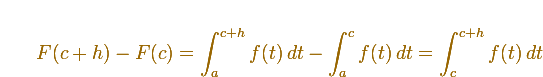If h is small enough (to be more precise, here you can use the mean-value theorem)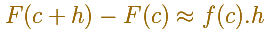Dividing by h: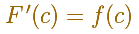If f has better properties, if f is continuous at all points in [a,b], then F is differentiable at all points in [a,b] andor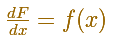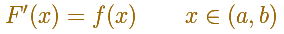We consider an indefinite integral function F (dragging the lower limit of integration you get different integral functions):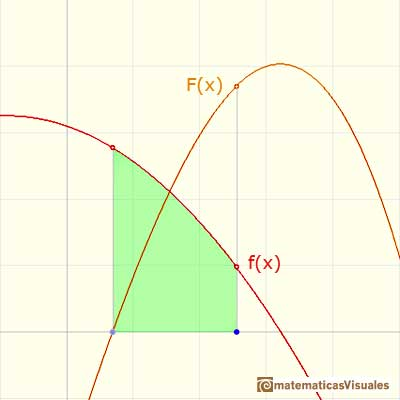At a point we differentiate this function F (graphically, this is the slope of the tangent line):And then:This FTC tell us that every continuous function has an antiderivative and shows how to construct one using an indefinite integral. Even those non-differentiable functions with "corners" such as absolute value has an antiderivative.

Sometimes the problem is how to find an antiderivative of a function: given a function f(x), find a function F(x) such that F'(x) = f(x).

An important case is when we want to integrate a function that has an antiderivative (a primitive). It is to say, you know a function f and you want to integrate f' (or you have to integrate f' and you can find an antiderivative f). In this case, we see the function to be integrate as a rate of change and the integral as an accumulator of this change (example: the integral of the velocity is the distance).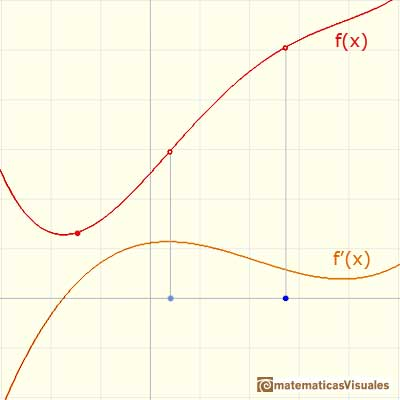You define an integral function F (but now we are integrating f'):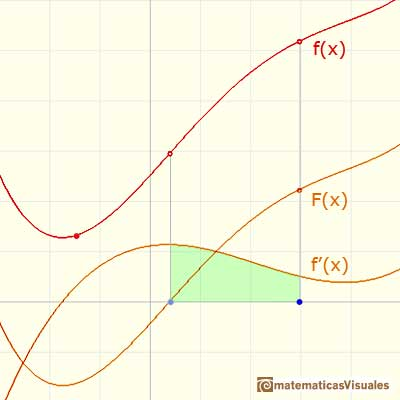And then F is and antiderivative of f', it is to say: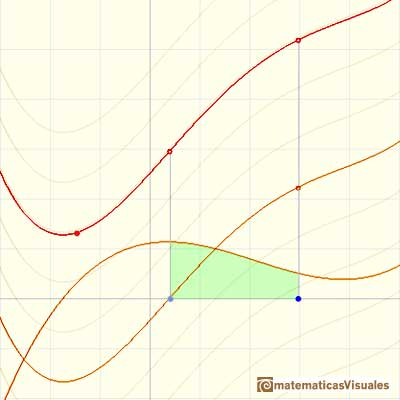And you can see thatOne step more and we are going to have the Second Fundamental Theorem of Calculus (or the evaluation part of the theorem).

REFERENCES

Michael Spivak, Calculus, Third Edition, Publish-or-Perish, Inc.
Tom M. Apostol, Calculus, Second Edition, John Willey and Sons, Inc.
Otto Toeplitz, The Calculus, a genetic approach, The University of Chicago Press, 1963 (p. 95-99).
Kenneth A. Ross, Elementary Analysis: The Theory of Calculus, Springer-Verlag New York Inc., 1980 (p. 190).
Serge Lang, A First Course in Calculus, Third Edition, Addison-Wesley Publishing Company.
David M. Bressoud, Historical Reflections on Teaching the Fundamental Theorem of Calculus, American Mathematical Monthly 118 (2011).
Jorge M. López Martínez and Omar A. Hernández Rodríguez,Teaching the Fundamental Theorem of Calculus: A Historical Reflection in MathDL.It is easy to calculate the area under a straight line. This is the first example of integration that allows us to understand the idea and to introduce several basic concepts: integral as area, limits of integration, positive and negative areas.To calculate the area under a parabola is more difficult than to calculate the area under a linear function. We show how to approximate this area using rectangles and that the integral function of a polynomial of degree 2 is a polynomial of degree 3.Archimedes show us in 'The Method' how to use the lever law to discover the area of a parabolic segment.The integral of power functions was know by Cavalieri from n=1 to n=9. Fermat was able to solve this problem using geometric progressions.If the derivative of F(x) is f(x), then we say that an indefinite integral of f(x) with respect to x is F(x). We also say that F is an antiderivative or a primitive function of f.The integral concept is associate to the concept of area. We began considering the area limited by the graph of a function and the x-axis between two vertical lines.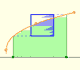Monotonic functions in a closed interval are integrable. In these cases we can bound the error we make when approximating the integral using rectangles.If we consider the lower limit of integration a as fixed and if we can calculate the integral for different values of the upper limit of integration b then we can define a new function: an indefinite integral of f.The derivative of a lineal function is a constant function.As an introduction to Piecewise Linear Functions we study linear functions restricted to an open interval: their graphs are like segments.A piecewise function is a function that is defined by several subfunctions. If each piece is a constant function then the piecewise function is called Piecewise constant function or Step function.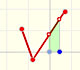A continuous piecewise linear function is defined by several segments or rays connected, without jumps between them.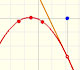The derivative of a quadratic function is a linear function, it is to say, a straight line.The derivative of a cubic function is a quadratic function, a parabola.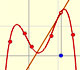Lagrange polynomials are polynomials that pases through n given points. We use Lagrange polynomials to explore a general polynomial function and its derivative.Two points determine a stright line. As a function we call it a linear function. We can see the slope of a line and how we can get the equation of a line through two points. We study also the x-intercept and the y-intercept of a linear equation.Power with natural exponents are simple and important functions. Their inverse functions are power with rational exponents (a radical or a nth root)Polynomials of degree 2 are quadratic functions. Their graphs are parabolas. To find the x-intercepts we have to solve a quadratic equation. The vertex of a parabola is a maximum of minimum of the function.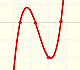Polynomials of degree 3 are cubic functions. A real cubic function always crosses the x-axis at least once.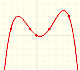We can consider the polynomial function that passes through a series of points of the plane. This is an interpolation problem that is solved here using the Lagrange interpolating polynomial.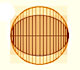In his book 'On Conoids and Spheroids', Archimedes calculated the area of an ellipse. We can see an intuitive approach to Archimedes' ideas.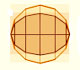In his book 'On Conoids and Spheroids', Archimedes calculated the area of an ellipse. It si a good example of a rigorous proof using a double reductio ad absurdum.Kepler used an intuitive infinitesimal approach to calculate the area of a circle.Kepler was one mathematician who contributed to the origin of integral calculus. He used infinitesimal techniques for calculating areas and volumes.Studying the volume of a barrel, Kepler solved a problem about maxima in 1615.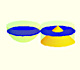Using Cavalieri's Principle we can calculate the volume of a sphere.